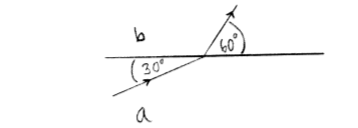Problem: A ray of light traveling in material a strikes the interface between materials  a and b and refracts into material b. In material a, the light makes an angle of 30° with respect to the surface of material a and in material b, it makes an angle of 60° with respect to the surface of material  b. Which of the following statements is correct? (a) The light travels faster in material b than in material a. (b) The light travels slower in material b than in material a. (c) The speed of the light is the same in both materials.

92% (6 ratings)
Problem Details

A ray of light traveling in material a strikes the interface between materials  a and b and refracts into material b. In material a, the light makes an angle of 30° with respect to the surface of material a and in material b, it makes an angle of 60° with respect to the surface of material  b. Which of the following statements is correct?

(a) The light travels faster in material b than in material a.

(b) The light travels slower in material b than in material a.

(c) The speed of the light is the same in both materials.